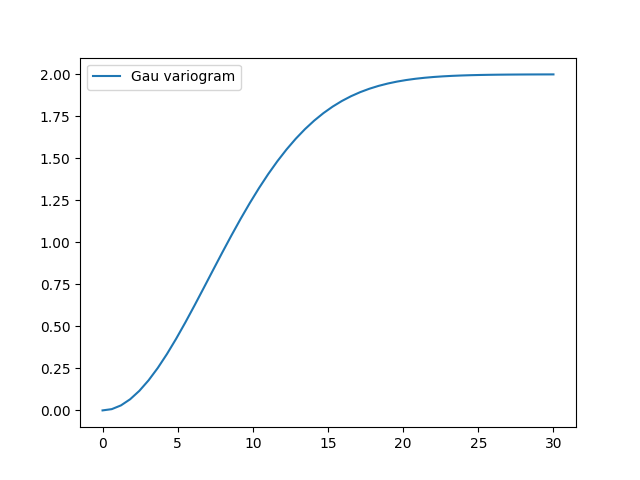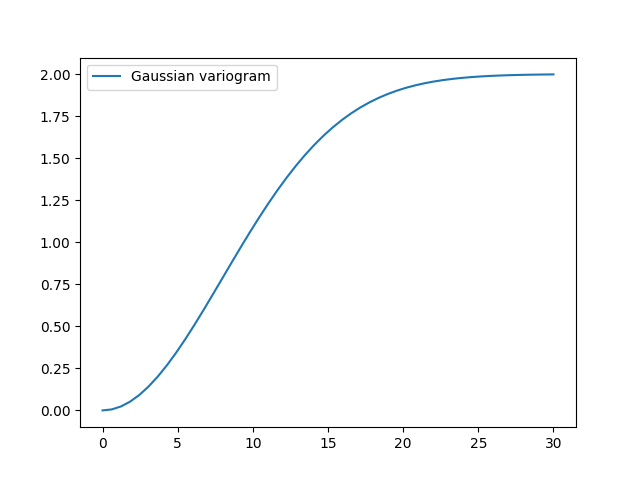# Introductory example¶

Let us start with a short example of a self defined model (Of course, we provide a lot of predefined models [See: `gstools.covmodel`], but they all work the same way). Therefore we reimplement the Gaussian covariance model by defining just the “normalized” correlation function:

```import numpy as np
import gstools as gs

# use CovModel as the base-class
class Gau(gs.CovModel):
def cor(self, h):
return np.exp(-h ** 2)
```

Here the parameter `h` stands for the normalized range `r / len_scale`. Now we can instantiate this model:

```model = Gau(dim=2, var=2.0, len_scale=10)
```

To have a look at the variogram, let’s plot it:

```model.plot()
```This is almost identical to the already provided `Gaussian` model. There, a scaling factor is implemented so the len_scale coincides with the integral scale:

```gau_model = gs.Gaussian(dim=2, var=2.0, len_scale=10)
gau_model.plot()
```## Parameters¶

We already used some parameters, which every covariance models has. The basic ones are:

• dim : dimension of the model
• var : variance of the model (on top of the subscale variance)
• len_scale : length scale of the model
• nugget : nugget (subscale variance) of the model

These are the common parameters used to characterize a covariance model and are therefore used by every model in GSTools. You can also access and reset them:

```print("old model:", model)
model.dim = 3
model.var = 1
model.len_scale = 15
model.nugget = 0.1
print("new model:", model)
```

Out:

```old model: Gau(dim=2, var=2.0, len_scale=10, nugget=0.0, anis=[1.], angles=[0.])
new model: Gau(dim=3, var=1.0, len_scale=15, nugget=0.1, anis=[1. 1.], angles=[0. 0. 0.])
```

Note

• The sill of the variogram is calculated by `sill = variance + nugget` So we treat the variance as everything above the nugget, which is sometimes called partial sill.
• A covariance model can also have additional parameters.

Total running time of the script: ( 0 minutes 0.244 seconds)

Gallery generated by Sphinx-Gallery